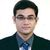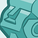# QlikView App Dev

Discussion Board for collaboration related to QlikView App Development.

Announcements
Now Live: Qlik Sense SaaS Simplified Authoring – Analytics Creation for Everyone: READ DETAILS
cancel
Showing results for
Did you mean:Creator III

## For Next Loop

 =(if(Month(LoadDate_Active)>1,Let c=0for a = Month(LoadDate_Active) to 1 step -1Let c = \$(c) + sum({\$} bal)next\$(c),sum({\$} bal)))/(100*1000000)

Shumail

1 Solution

Accepted SolutionsMVP

I may be missing something but is this not a simple pivot with Months as the dimension along the top and then Set Analysis in the expression to limit to the max year and months prior to the max selected?

Something like:

sum({<Year={\$(vMaxYear)},Date={'<=\$(vMaxDate)'},Month=>}Value)

I've also attached an example that may help.

By extending the example to also be aware of the Prior year you could put in a YTD prior year comparison with variances.

18 RepliesSpecialist III

Where are you using this expression?

Set expressions do not work in load script and for ...next loop is not available in chart expressions.Creator III
Author

Is there any alternate of "For..Next" loop in chart expression?

Also

Is there ne matrix for all these details I mean something like

 Load Script Expression Macro (vbscript) For Next Loop Yes No Yes Do While Loop Yes No Yes Set No Yes Yes

ShumailMVP

Hi,

As krishnamoorthy said, the looping can not be done in expression.

There is no matrix available like you shown.

Why you want to use for loop in expression.

Provide us your problem definition, may be there are some other solution available.

Regards,

Kaushik Solanki

Regards,
KaushikCreator III
Author

Actually I am looking for the sum of customer balances by year like if i select May-2011 then expression can sum all the values in 2011 before and in may-2011 excluding june-2011 onward. similarly with every month.

I think I have to apply many nested If conditions like this

=(

,sum({\$<AIF = {P}, {\$<MonthName_ID = {'\$(=MonthName(LoadDate))'}, Bucket_MOM = {0,1,2,3,4,5,6}>} bal)

sum({\$<AIF = {P}, {\$<MonthName_ID = {'\$(=MonthName(LoadDate))'}, Bucket_MOM = {0,1,2,3,4,5,6}>} bal)

+

sum({\$<AIF = {P}, {\$<MonthName_ID = {'\$(=MonthName(LoadDate,-1))'}, Bucket_MOM = {0,1,2,3,4,5,6}>} bal)

sum({\$<AIF = {P}, {\$<MonthName_ID = {'\$(=MonthName(LoadDate))'}, Bucket_MOM = {0,1,2,3,4,5,6}>} bal)

+

sum({\$<AIF = {P}, {\$<MonthName_ID = {'\$(=MonthName(LoadDate,-1))'}, Bucket_MOM = {0,1,2,3,4,5,6}>} bal)

+

sum({\$<AIF = {P}, {\$<MonthName_ID = {'\$(=MonthName(LoadDate,-2))'}, Bucket_MOM = {0,1,2,3,4,5,6}>} bal)

..

...

.... till the twelve months

sum({\$<AIF = {P}, {\$<MonthName_ID = {'\$(=MonthName(LoadDate))'}, Bucket_MOM = {0,1,2,3,4,5,6}>} bal)

+

sum({\$<AIF = {P}, {\$<MonthName_ID = {'\$(=MonthName(LoadDate,-1))'}, Bucket_MOM = {0,1,2,3,4,5,6}>} bal)

+

..

...

....

sum({\$<AIF = {P}, {\$<MonthName_ID = {'\$(=MonthName(LoadDate,-11))'}, Bucket_MOM = {0,1,2,3,4,5,6}>} bal)

)

)

)

)

/(100*1000000)

ShumailMVP

Hi,

Let me say what i understood from this is that,

when you select May-2011, you want data from Jan-2011 till May-2011.

Am i right.

Regards,

Kaushik Solanki

Regards,
KaushikEmployee

Generally it is really not recommende to use to many nested IF statements, since this can have serious impact on the application performance. You are much better of if you can find a solution using set analysis and/or Aggr().

An other option can be to do some additonal calculations in the load script, so that parts are statically precalculated and only needs to be sumed in the expression. This would be of some performance gain in case you run larger data volumes or a slower machine.

It would be easier to advise on a good approach if you attach a sample of you solution, so that the data model can be evaluated.Creator III
Author

Yes exactly you are right kaushik!

Thanks for the reply Toni, I am attaching one sample of my project. I am having problem on disabled field COF Final... Please see it on expression tab and if you have any solution then let me know... Thanks in Advance.

ShumailContributor

I have a solution but i cant seem to upload to the forum.

send me  your email and i wil send the solution.

Try somehting like this in your script

I use it  together with a calendar

I used these with set analysis to calculate some expressions in my chart(i.e. YTD,MTD,WTD...etc)

IF(M>3,Y+1,Y)as FinYear,

YearName(D,0,4) as FinPeriod,MVP

I may be missing something but is this not a simple pivot with Months as the dimension along the top and then Set Analysis in the expression to limit to the max year and months prior to the max selected?

Something like:

sum({<Year={\$(vMaxYear)},Date={'<=\$(vMaxDate)'},Month=>}Value)

I've also attached an example that may help.

By extending the example to also be aware of the Prior year you could put in a YTD prior year comparison with variances.